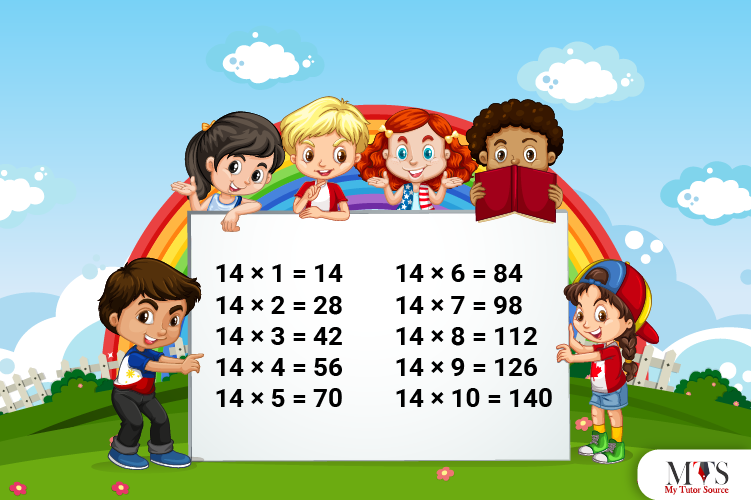# Multiplication Table of 14 – Tips to Memorize 14 Times Table & Example QuestionsMultiplication tables are the tool to promote number sense and develop key skills. It’s the responsibility of every school teacher or private math tutor to make memorizing multiplication tables a rewarding and enjoyable subject to master. It helps students solve mathematical problems within seconds and get rid of time-consuming ways. The process of learning 14 times is easy. In this article, we have shared a table of 14, a method to read and write, and shortcuts to learn quickly. Moreover, stick around to find the solved example questions for practice purposes.

## Table of 14

Students preparing for UPSC, GRE, SAT, and other competitive exams must memorize a table of 15 to perform quick calculations. Below, we have provided the 14 times table to its 20 multipliers. Also, this is the way we write the multiplication table of 14. Check it out

14 x 1 = 14
14 x 2 = 28
14 x 3 = 42
14 x 4 = 56
14 x 5 = 70
14 x 6 = 84
14 x 7 = 98
14 x 8 = 112
14 x 9 = 126
14 x 10 = 140
14 x 11 = 154
14 x 12 = 168
14 x 13 = 182
14 x 14 = 196
14 x 15 = 210
14 x 16 = 224
14 x 17 = 238
14 x 18 = 252
14 x 19 = 266
14 x 20 = 280

## Method to Read Table of 14

It requires a high level of dedication, encouragement, and patience to memorize each multiplication table at your fingertips. Among plenty of reading methods, we have provided a pretty easy method to read the multiplication table of 14. You can use the following to read and memorize the 14 times table:

Fourteen ones are fourteen (14)
Fourteen twos are twenty-eight (28)
Fourteen threes are forty-two (42)
Fourteen fours are fifty-six (56)
Fourteen fives are seventy (70)
Fourteen sixes are eighty-four (84)
Fourteen sevenths are ninety-eight (98)
Fourteen eighths are one hundred and twelve (112)
Fourteen nines are one hundred and twenty-six (126)
Fourteen tens are one hundred and forty (140)
Fourteen elevens are one hundred and fifty-four (154)
Fourteen twelves are one hundred and sixty-eight (168)
Fourteen thirteens are one hundred and eighty-two (182)
Fourteen fourteens are one hundred and ninety-six (196)
Fourteen fifteenths are two hundred and ten (210)
Fourteen sixteenths are two hundred and twenty-four (224)
Fourteen seventeens are two hundred and thirty-eight (238)
Fourteen eighteenths are two hundred and fifty-two (252)
Fourteen nineteens are two hundred and sixty-six (266)
Fourteen twenties are two hundred and eighty (280)

## Tips for Memorizing Multiplication Table of 14

The table of 14 doesn’t come with rules or an easy pattern which is why it is hard for students to memorize quickly compared to other multiplication tables. However, a few tips can help you memorize the results of the 14 times table, such as using the repeated addition method or doubling the table of 4.

The addition method is a bit risky because adding large numbers sometimes gets out of hand for students. Therefore, we would advise you to use double the table of 4 tips. Here is how:

Use a 4 times table and add natural numbers (multipliers) to the tens’ digit of its product to generate the results of the 14 times table.

### Example Question of Table of 14

Question: Find the value of 14 times 7 plus 14 times 9?

Solution:

Given that,
14 times 7 plus 14 times 9
In mathematical form,
= 14 x 7 + 14 x 9
Using 14 times table
= 98 + 126
= 224
Hence, the value of 14 times 7 plus 14 times 9 is 224

Question: Using the table of 14, find how many fourteens are in 56?

#### Solution:

Let p be the number,
⇒ p x 14 = 56
⇒ p = 56/14
⇒ p = 4
Hence, there are 4 fourteens in 56.

Question: Today is a chocolate day at school, and the teacher wants to distribute 2 chocolates to each student. If a class has 14 students, find how many chocolates he would need for distribution? Find the number of chocolates using the 14 times table.

#### Solution:

Given that,
Number of chocolates for each student = 2
Number of students = 14
Using 14 times table,
⇒ 14 x 2 = 28
Hence, the teacher needs a total of 28 chocolates to distribute

Question: Write a multiplication table of 14 from 2 to 8.

14 x 2 = 28
14 x 3 = 42
14 x 4 = 56
14 x 5 = 70
14 x 6 = 84
14 x 7 = 98
14 x 8 = 112

Question: Fill in the blanks using 7 multiplication table

14 x ___ = 56

___ x 6 = 84

___ x ___ = 112

14 x ___ = 98

___ x ___ = 42

14 x ___ = 126

___ x 13 = 182

___ x ___ = 252

Question: How many dollars do I need to buy 7 flowers if each flower costs \$14?

Solution:

Given that,
Total flowers = 7
Cost of each flower = \$14
Using multiplication table of 14
⇒ 7 x 14 = 98
Hence, you would need \$98 to buy 7 flowers.

### Find Top Tutors in Your AreaWith over 3 years of experience in teaching, Chloe is very deeply connected with the topics that talk about the educational and general aspects of a student's life. Her writing has been very helpful for students to gain a better understanding of their academics and personal well-being. I’m also open to any suggestions that you might have! Please reach out to me at chloedaniel402 [at] gmail.com